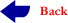MATH 101 - Calculus I (4-0-4)

 Homework FirstMajor Solution Quizzes Solution Q#7 Second Major Solution Final Solution Syllabus

New Second Major Exam

Syllabus

## Catalog Description

Limits and continuity of functions of a single variable.  Differentiability. Techniques of differentiation.   Implicit differentiation.  Local extrema, first and second derivative tests for local extrema.  Concavity and inflection points.  Curve sketching.  Applied extrema problems.  The Mean Value Theorem and applications.

Prerequisite: One year preparatory mathematics or its equivalent

Textbook:  Calculus, A New Horizon by Howard Anton, sixth edition (1999).

 Week(s) Dates Sec. Topics 1 June 18 – 19 2.1 Limits (An intuitive Introduction) 2 June  22 – 27 2.2 2.3 2.4 2.5 Limits (Computational Techniques) Limits (Discussed more rigorously) Continuity Limits and Continuity of Trigonometric Functions 3 June 29 –July 3 3.1 3.2 3.3 3.4 Tangent Lines and Rates of Change The Derivative Techniques of Differentiation Derivatives of Trigonometric Functions 4 July 5 – July 10 3.5 3.6 4.1 4.2 The Chain Rule Local Linear Approximation; Differentials Inverse Functions Logarithmic and Exponential Functions 5 July12 –July 17 4.3 4.4 4.5 4.6 Implicit Differentiation Derivatives of Logarithmic and Exponential Functions Derivatives of Inverse Trigonometric Functions Related Rates 6 July 19 – July 24 4.7 5.1 5.2 L’Hopital’s Rule; Indeterminate Forms Increasing, Decreasing, and Concavity Relative Extrema; First and Second Derivative Tests 7 July 27 – July 31 5.3 6.1 6.2 6.3 Applying Technology and the Tools of Calculus Absolute Maxima and Minima Applied Maximum and Minimum Problems Rectilinear Motion 8 Aug.3 – Aug. 7 6.4 6.5 Newton’s Method Rolle’s Theorem; Mean-Value Theorem Review 9 Aug.10 –Aug. 12 Final exams

Grading Policy: First Major 15 points, Second Major 15 points, Homework 5 points, Quizzes and Matlab quiz 20 points, Final 35 points.